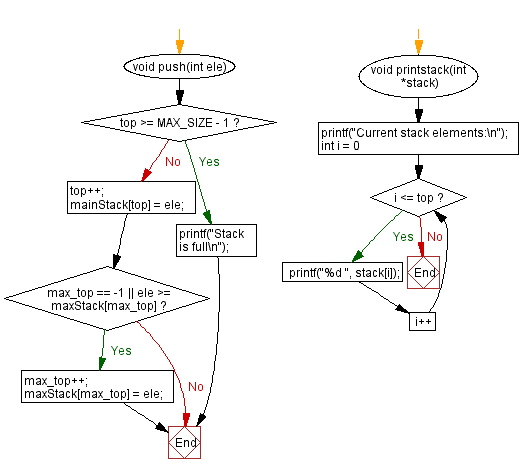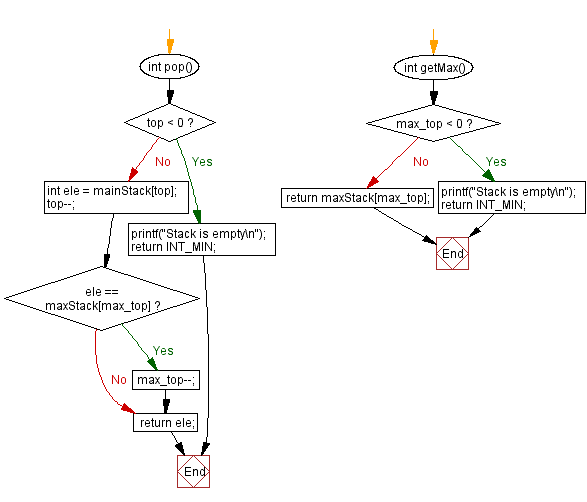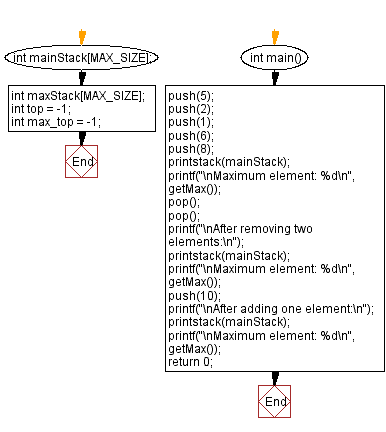﻿ C - Find the maximum element in a stack

# C Exercises: Find the maximum element in a stack

## C Stack: Exercise-12 with Solution

Write a C program to find the maximum element in a stack.

Sample Solution:

C Code:

``````#include <stdio.h>
#include <stdlib.h>
#include <limits.h>

#define MAX_SIZE 100

int mainStack[MAX_SIZE];
int maxStack[MAX_SIZE];
int top = -1;
int max_top = -1;

void push(int ele) {
if (top >= MAX_SIZE - 1) {
printf("Stack is full\n");
return;
}

top++;
mainStack[top] = ele;

if (max_top == -1 || ele >= maxStack[max_top]) {
max_top++;
maxStack[max_top] = ele;
}
}

int pop() {
if (top < 0) {
printf("Stack is empty\n");
return INT_MIN;
}

int ele = mainStack[top];
top--;
if (ele == maxStack[max_top]) {
max_top--;
}
return ele;
}
int getMax() {
if (max_top < 0) {
printf("Stack is empty\n");
return INT_MIN;
}

return maxStack[max_top];
}

void printstack(int *stack)
{
printf("Current stack elements:\n");
for (int i = 0; i <= top; i++) {
printf("%d ", stack[i]);
}
}

int main() {
push(5);
push(2);
push(1);
push(6);
push(8);
printstack(mainStack);
printf("\nMaximum element: %d\n", getMax());
pop();
pop();
printf("\nAfter removing two elements:\n");
printstack(mainStack);
printf("\nMaximum element: %d\n", getMax());
push(10);
printstack(mainStack);
printf("\nMaximum element: %d\n", getMax());
return 0;
}
```
```

Sample Output:

```Current stack elements:
5 2 1 6 8
Maximum element: 8

After removing two elements:
Current stack elements:
5 2 1
Maximum element: 5

Current stack elements:
5 2 1 10
Maximum element: 10
```

Flowchart:C Programming Code Editor:

Have another way to solve this solution? Contribute your code (and comments) through Disqus.

What is the difficulty level of this exercise?

Test your Programming skills with w3resource's quiz.

﻿

## C Programming: Tips of the Day

C Programming - How do you pass a function as a parameter in C?

Declaration

A prototype for a function which takes a function parameter looks like the following:

```void func ( void (*f)(int) );
```

This states that the parameter f will be a pointer to a function which has a void return type and which takes a single int parameter. The following function (print) is an example of a function which could be passed to func as a parameter because it is the proper type:

```void print ( int x ) {
printf("%d\n", x);
}
```

Function Call

When calling a function with a function parameter, the value passed must be a pointer to a function. Use the function's name (without parentheses) for this:

```func(print);
```

would call func, passing the print function to it.

Function Body

As with any parameter, func can now use the parameter's name in the function body to access the value of the parameter. Let's say that func will apply the function it is passed to the numbers 0-4. Consider, first, what the loop would look like to call print directly:

```for ( int ctr = 0 ; ctr < 5 ; ctr++ ) {
print(ctr);
}
```

Since func's parameter declaration says that f is the name for a pointer to the desired function, we recall first that if f is a pointer then *f is the thing that f points to (i.e. the function print in this case). As a result, just replace every occurrence of print in the loop above with *f:

```void func ( void (*f)(int) ) {
for ( int ctr = 0 ; ctr < 5 ; ctr++ ) {
(*f)(ctr);
}
}
```

Ref : https://bit.ly/3skw9Um